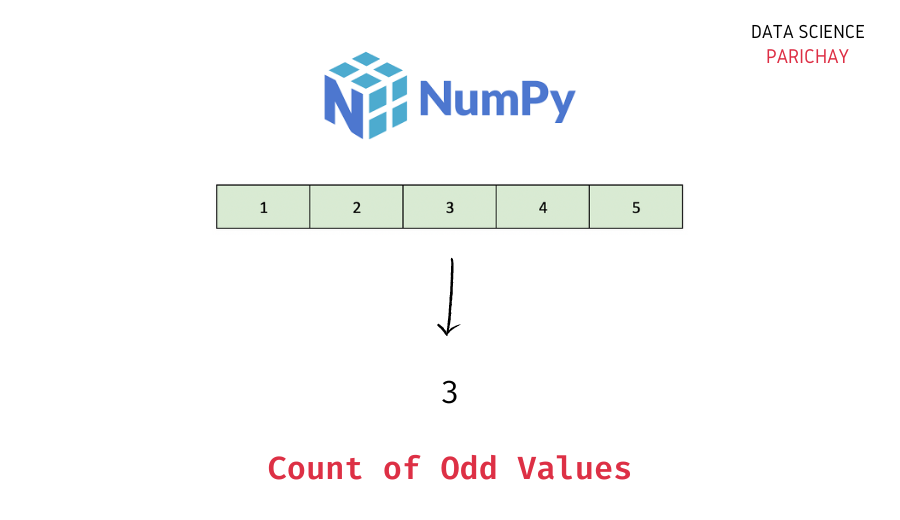# Numpy – Count of Odd Values in Array

In this tutorial, we will look at how to count the odd values in a Numpy array with the help of some examples.

## Steps to get the count of odd values in a Numpy array

In general, to find the count of values in a Numpy array that satisfy the given condition, you can –

1. Use boolean indexing to filter the array for only the values that satisfy the condition.
2. Calculate the length of the filtered array from step 1.

Thus, first, filter the Numpy array to contain only the odd values and then find its length to get the required count.

Let’s now look at a step-by-step example.

### Step 1 – Create a Numpy array

First, we will create a Numpy array that we will be using throughout this tutorial.

```import numpy as np

# create a numpy array
ar = np.array([1, 2, 3, 4, 5, 6, 7])
# display the array
print(ar)```

Output:

`[1 2 3 4 5 6 7]`

Here, we used the `numpy.array()` function to create a one-dimensional Numpy array containing some numbers.

### Step 2 – Filter the array using a boolean expression

To get all the values from a Numpy array that are odd, filter the array using boolean indexing.

📚 Data Science Programs By Skill Level

Introductory

Intermediate ⭐⭐⭐

🔎 Find Data Science Programs 👨‍💻 111,889 already enrolled

Disclaimer: Data Science Parichay is reader supported. When you purchase a course through a link on this site, we may earn a small commission at no additional cost to you. Earned commissions help support this website and its team of writers.

First, we will specify our boolean expression, `ar % 2 != 0` and then use the boolean array resulting from this expression to filter our original array.

Let’s get all the odd values in the above array.

```# odd values in the array ar
ar_filtered = ar[ar % 2 != 0]
print(ar_filtered)```

Output:

`[1 3 5 7]`

We get all the values in the array `ar` that are odd.

### Step 3 – Get the length of the filtered array

To get the count of values that satisfy the given condition (whether it’s odd or not) find the length of the resulting filtered array from step 2 using the Python built-in `len()` function.

```# length of the filtered array
print(len(ar_filtered))```

Output:

`4`

We get the count of odd values in the array `ar` as 4.

We can combine the code from the last two steps into a single line of code.

```# count of odd values in the array ar
print(len(ar[ar % 2 != 0]))```

Output:

`4`

We get the same result as above and we removed the extra variable `ar_filtered`.

In this tutorial, we looked at how to count odd values in a Numpy array. Note that in this method we’re not counting unique elements that are odd, rather we’re counting all values in an array that are odd (which may include duplicates depending on the array).

You might also be interested in –

•